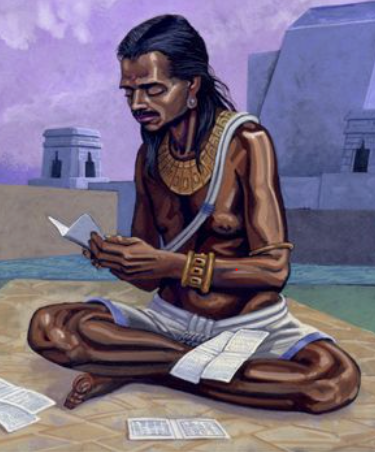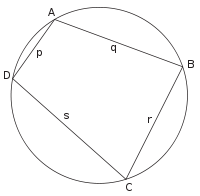Mathematics
Brahmagupta | Great Indian Mathematician
33k views

 1 Introduction 2 Who is Brahmagupta? 3 Works of Brahmagupta 4 Brahmagupta formula 5 Brahmagupta achievements 6 Summary 7 FAQs

22 September 2020

Read time: 3 minutes

## Introduction

In India, it is second nature to consult an astrologer who suggests an auspicious time of Muhurat for an important event. In other words, Astrology and Astronomy is a part of our life. Our calendar both the solar and the lunar calendar accurately calculate the festivals, moon phases, eclipses and many other happenings in not just our solar system, but also the cosmos or the Universe. What is impressive is that our ancient Astronomers and Astrologers used mathematics to calculate the auspicious timing for important events in our life.

One thing our ancient scientists were aware of was that there is an order/ logic in this huge expanse and vastness. It is this realization that led to the discoveries in Mathematics.

## Who is Brahmagupta?Brahmagupta ( 597- 668AD) was one such genius Astronomer - Mathematician. His father Jisnugupta was an Astrologer in the city of Bhinmal ( Rajasthan). Brahmagupta too considered himself an Astronomer however today he is remembered for his huge contributions to the field of Mathematics. By his admission, he did Mathematics or solved problems for pleasure!

Ujjain was the centre of Ancient Indian mathematical astronomy. Brahmagupta was the director of this centre. Brahmagupta wrote many textbooks for mathematics and astronomy while he was in Ujjain. These include ‘Durkeamynarda’ (672), ‘Khandakhadyaka’ (665), ‘Brahmasphutasiddhanta’ (628) and ‘Cadamakela’ (624). The ‘Brahmasphutasiddhanta’ meaning the ‘Corrected Treatise of Brahma’ is one of his well-known works.

## Works of Brahmagupta

Brahmagupta, like all scholars in those times, wrote in elliptical verse.

Brahmasphutasiddhanta ((Brahma’s Correct System of Astronomy, or The Opening of the Universe.) written in 628 was his most famous work. This book has twenty-five chapters and a total of 1008 stanzas. Historians believe that the first ten were originally written by Brahmagupta because they are arranged like the typical mathematical astronomy texts in that period. It covers mean longitudes of the planets; true longitudes of the planets; the three problems of diurnal rotation; lunar eclipses; solar eclipses; risings and settings; the moon's crescent; the moon's shadow; conjunctions of the planets with each other; and conjunctions of the planets with the fixed stars.

The remaining fifteen chapters seem to form a second work which is a major addendum to the original treatise.

Brahmasphutasiddhanta is the earliest known text that treated zero as a number. The Greeks and Romans merely used symbols, and the Babylonians used a shell to represent nothing.

He gave the concept of positive numbers which he called wealth or dhan and negative numbers which he called debt or ऋण

He wrote the rules as follows:

 A debt minus zero is a debt. A fortune minus zero is a fortune. Zero minus zero is a zero. A debt subtracted from zero is a fortune. A fortune subtracted from zero is a debt. The product of zero multiplied by a debt or fortune is zero. The product of zero multiplied by zero is zero. The product or quotient of two fortunes is one fortune. The product or quotient of two debts is one fortune. The product or quotient of a debt and a fortune is a debt. The product or quotient of a fortune and a debt is a debt.

This was a revolution as most people dismissed the possibility of a negative number thereby proving that quadratic equations (of the type $$\rm{}x2 + 2 = 11,$$ for example) could, in theory, have two possible solutions, one of which could be negative, because $$32 = 9$$ and $$-32 = 9$$. Brahmagupta went yet further by considering systems of simultaneous equations (set of equations containing multiple variables) and solving quadratic equations with two variables

An example from Brahmasphutasiddhanta

Five hundred drammas were loaned at an unknown rate of interest. The interest on the money for four months was loaned to another at the same rate of interest and amounted in ten months to $$78$$ drammas. Give the rate of interest.

## Brahmagupta Formula

Brahmagupta found the formula for cyclic quadrilaterals though he did not focus on the cyclic character of the figure. Given the lengths of the sides of any cyclic quadrilateral, Brahmagupta gave an approximate and an exact formula for the figure's area.$$\text{Area}=\sqrt{{(S-p)}{(S-q)}{(S-r)}{(S-s)}}\\Where \text { S}=\frac{(p+q+r+s)}{2}$$

His second book The Khandakhadyaka - 665 AD has eight chapters. This book too details longitudes of the planets; the three problems of diurnal rotation; lunar eclipses; solar eclipses; risings and settings; the moon's crescent; and conjunctions of the planets.

What stands out as a mathematical genius in this work is the interpolation formula he uses to compute values of sines.

## Brahmagupta achievements

• Brahmagupta defined the properties of the number zero, which was crucial for the future of mathematics and science. Brahmagupta enumerated the properties of zero as:

★ When a number is subtracted from itself, we get a zero

★ Any number divided by zero will have the answer as zero

★ Zero divided by zero is equal to zero

• Discovered the formula to solve quadratic equations.

• Discovered the value of pi ( 3.162….) almost accurately. He put the value 0.66% higher than the true value. ( 3.14)

• With calculations, he indicated that Earth is nearer to the moon than the sun.

• Found a formula to calculate the area of any four-sided figure whose corners touch the inside of a circle.

• Calculated the length of a year is 365 days 6 hours 12 minutes 9 seconds.

• Brahmagupta talked about ‘gravity.’ To quote him, ‘Bodies fall towards the earth as it is in the nature of the earth to attract bodies, just as it is in the nature of water to flow.’

• Proved that the Earth is a sphere and calculated its circumference to be around 36,000 km (22,500 miles).

• Brahmagupta established rules for working with positive and negative numbers, such as:

Ø Negative $$+$$ Negative number $$=$$ Negative number

Ø Subtracting a Negative from a positive number is the same as adding the two numbers.

Ø Negative X Negative number$$=$$ Positive number.

Ø Positive number ÷ Negative number $$=$$ Negative number.

## Summary

Indian philosophy reiterates that we are a small part of a Brahmand, the cosmos or the universe. This humbling knowledge was perhaps the basis of the concept of a zero or a void because it came from a culture that conceived and acknowledged the idea of the infinite. A symbol $$(0)$$ denoting “nothing” was a part of Indian culture. This becomes particularly relevant as it indicates a vibrant, philosophical culture that recognised the power of nothingness and thus actually recognised the power of Mathematics and its role in the order of nothingness.

Although Brahmagupta thought of himself as an astronomer who did some mathematics, he is now mainly remembered for his contributions to mathematics. He was honoured by the title given to him by a fellow scientist Ganita Chakra Chudamani’ which is translated as ‘The gem of the circle of mathematicians’.

## What did brahmagupta discover?

Brahmadutta has a lot to his credit:

1. Brahmagupta gave the solution of the general linear equation in chapter eighteen of Brahmasphutasiddhānta.
2. He gave two equivalent solutions to the general quadratic equation.
3. Brahmagupta's Brahmasphuṭasiddhānta is the first book that provides rules for arithmetic manipulations that apply to zero and to negative numbers.
4. Brahmagupta's most famous result in geometry is his formula for cyclic quadrilaterals.

## Where was brahmagupta born?

Brahmagupta was born in the city of Bhinmal, Rajasthan.

## When was brahmagupta born?

Brahmagupta was born in 597 AD.

## When did brahmagupta die?

Brahmagupta passed away in 668 AD.